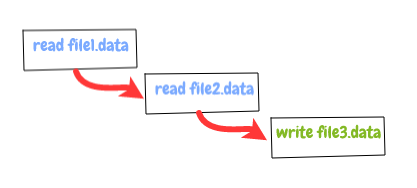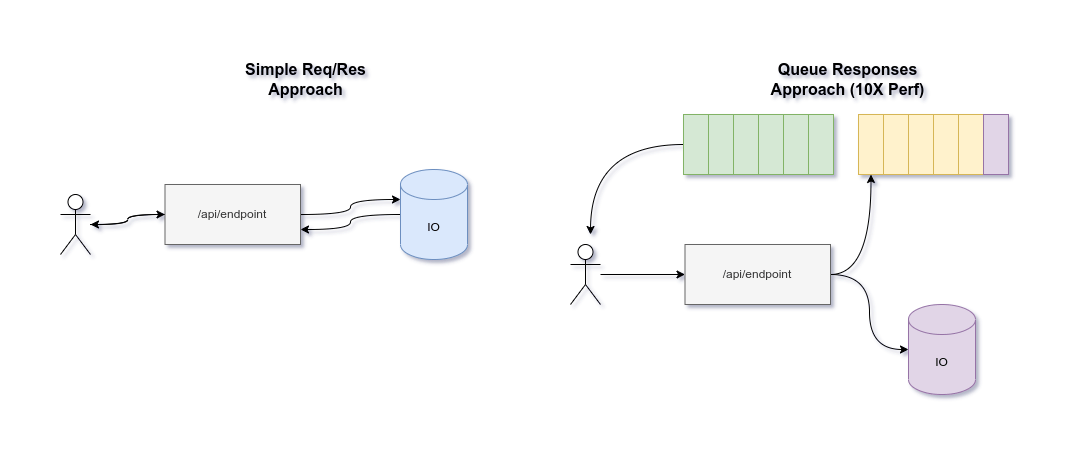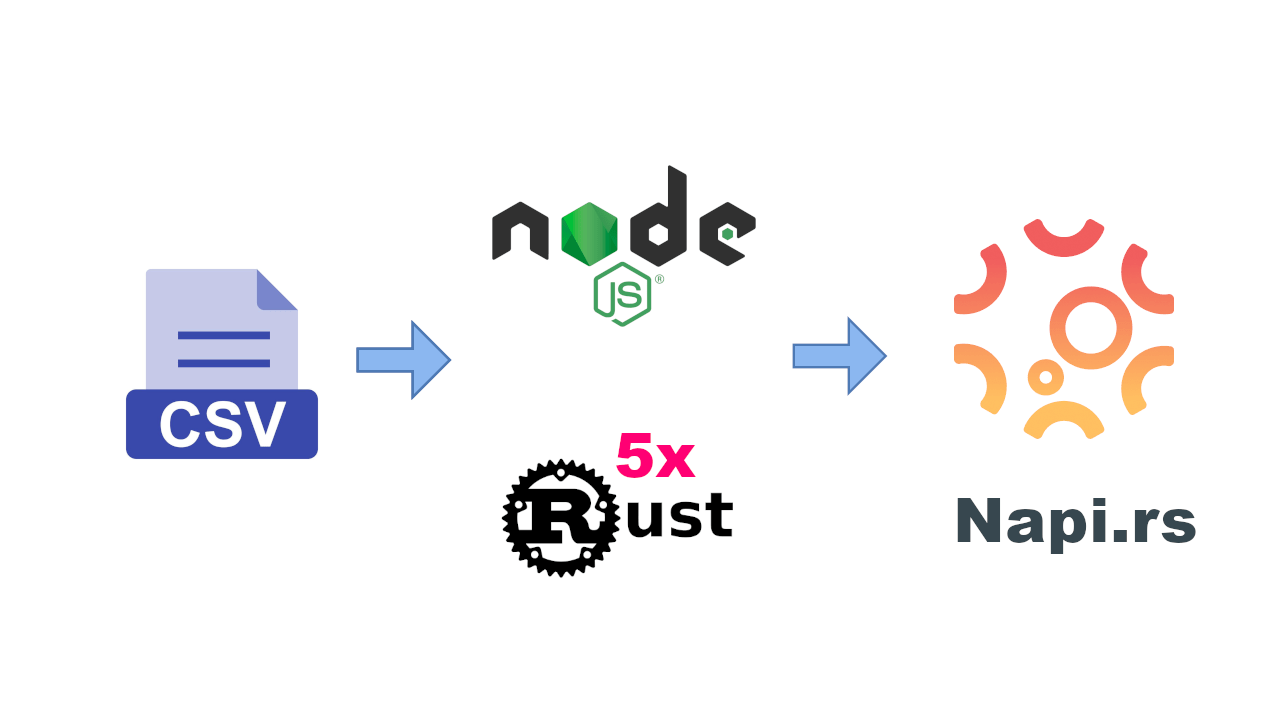alxolr

# Learning asynchronous programming in node.js with callbacks## Asynchrony

Asynchronous code is one of the most controversial aspects in node.js, but in practical terms we can safely associate the asynchrony word with parallel.

The nature of node.js is single threaded due to V8 javascript engine. But anyway we can run code in parallel. How this is possible ?

A good way to understand how this is possible, is by watching the following 2 videos on Event Loop.

## Asynchronous control flow

Assuming you understood how the event loop is working the next step is to tame this powerful benefit and create beautiful and extremely fast code.

Asynchronous control flow is nothing more than a series of patterns to deal with parallel programming in node.js.

## Run in sequence

Let's assume we have the following task, to concatenate the content of 2 files `file1.data`, `file2.data` and place it in the `file3.data`.
For the current task we will use the native `fs` module.

A very common solution will be to execute the code in asynchronous sequence as following.As you can see in the image we are waiting for the response of first operation `read file1.data` to finish then we read the second file `read file2.data` and after the second operation is done we are writing the result in the third file `file3.data`.

``````const fs = require('fs');
const assert = require('assert');

fs.readFile('file1.data', 'utf8', (err, file1) => {
assert.equal(err, null);

fs.readFile('file2.data', 'utf8', (err, file2) => {
assert.equal(err, null);

// we concatenate the content of the 2 files
const file3 = `\${file1}\n\${file2}`;

fs.writeFile('file3.data', file3, (err) => {
assert.equal(err, null);

console.log('Files are concatenated');
});
});
});``````

This is a common solution but not the best one.

A better solution will be to run the first 2 reads in parallel and then after they are done to write the result.

## Run in parallelIn order to run two tasks in parallel and wait for their callback to finish I need to introduce the concept of gate keeper;

A gate keeper is a callback attached to all the asynchronous functions and has the role to track how many parallel functions started and how many has finished, just like a real gate keeper with persons in a building.

``````const fs = require('fs');
const assert = require('assert');

function runFirstTwoInParallel(done) {
const files = [];
let pending = 0;

function gateKeeper() {
let order = pending;
pending++;
return (err, data) => {
pending--;
if (err) return done(err);
files[order] = data;

if (!pending) {
return done(null, files);
}
}
}
}

runFirstTwoInParallel((err, files) => {
assert.equal(err, null);
const [file1, file2] = files; // extract files from result
const file3 = `\${file1}\n\${file2}`;

fs.writeFile('file3.data', file3, (err) => {
assert.equal(err, null);
console.log('Files are concatenated');
});
});``````

As we can see the code suddenly got a bit complex, but not so difficult to understand. The most important function in our code is the `gateKeeper` function.

``````function gateKeeper() {
let order = pending; // 
pending++;  // 
return (err, data) => {
pending--; // 
if (err) return callback(err); //
files[order] = data; // 

if (!pending) { // 
return callback(null, files); // 
}
}
}``````

The gateKeeper() is synchronously executed function who returns the callback for the `fs.readFile`, but in meantime of the return it saves the order of the invocations  and count the number of pending callbacks 

As gateKeeper is synchronous at one point in time we will have `pending = 2` and no callback yet executed cause they are asynchronous. After the callback is executed we decrement the pending number .

If there are any errors, or one of the callbacks returned we continue the code execution by passing the ball to the `done` function . On point  we store the response of `fs.readFile` in the `files` array by using the `order` index created on .

On  if there are no more pending (pending is 0) callbacks then we execute our callback with no err, and the `files` array, which at this point should have the results of both asynchronous `fs.readFile` invocation.

Our code will be executed in the following manner:

`````` starts first gateKeeper()
order = 0;
pending = 1;

starts second gateKeeper()
order = 1;
pending = 2;

// 2 cases could follow:

// case 1
the callback for fs.readFile('file1.data') is first
order = 0;
pending = pending - 1 = 2 - 1 = 1;

the callback for fs.readFile('file2.data') is second
order = 1;
pending = pending - 1 = 1 - 1 = 0;

// case 2
the callback for fs.readFile('file2.data') is first
order = 1;
pending = pending - 1 = 2 - 1 = 1;

the callback for fs.readFile('file1.data') is second
order = 0;
pending = pending - 1 = 1 - 1 = 0;``````

## Run an array of tasks in parallel

Let's assume we have the task to save 100 objects in a random database.

``````function parallel(collection, callback) {
let pending = 0;
let results = [];

collection.forEach(obj => database.save(obj, gateKeeper()));

function gate(err, data) {
return callback(err, data);
}
}

function gateKeeper() {
let order = pending;
pending += 1;

return (err, result) => {
pending -= 1;
if (err) {
return gate(err);
}

results[order] = result;
if (!pending) {
gate(null, result);
}
}
}
}

const objects = new Array(100).fill(0)
.map((e, index) => ({
id: index + 1,
name: '<random name>',
createdAt: new Date()
}));

parallel(objects, (err, results) => {
if (err) console.log(err);

console.log('Objects were saved successfully.')
});``````

As we can see we have abstracted the boilerplate code of running multiple callbacks in parallel in a `parallel` function. Now we can easily create a module that can be reused in our projects. Running a big number of tasks in parallel is not always the best solution. Sometime we are in a need of limiting the number of calls. One of the examples can be the possibility of using a limiting api like google geolocation where you can make a certain amount of calls per second etc. In this type of situations we are in a need of a limited parallel queue.

### Run in a limited parallel queue

A limited parallel queue is an asynchronous pattern who have the role of limiting the number of tasks started in parallel to a specific `concurrency` by using a `queue`;

Let's investigate the following code, we will need a folder `limited-parallel-queue` with two files.
`parallel-queue.js` and `index.js`;

``````// parallel-queue.js
class ParallelQueue {
constructor(concurrency) { // 
this.concurrency = concurrency;
this.queue = []; // 
this.running = 0; // 
}

this.next(); // 
}

next() { // 
while(this.queue.length && this.running < this.concurrency ) {
this.running --;
this.next();
});
this.running ++;
}
}
}``````

From the code above we can observe that our `ParallelQueue` module is an `es6` class that has a `concurrency` parameters . Next thing we initialize an empty `queue`  which is just an empty array. And a running counter . The `push` function  is used to add the next task to the queue but more importantly to initiate the execution of some of the tasks that are in the queue by calling the `next()` function;

The source code of  the `next` function is relatively self explanatory. We start executing tasks in parallel if the running counter is bellow our concurrency threshold. And after each task finished we decrement the running counter and also triggering the `next` function to reiterate the process.

Next we will use the parallel queue in our `index.js` file.

``````// index.js
const ParallelQueue = require('./parallel-queue');
const queue = new ParallelQueue(2);

const array = new Array(100)
.fill(0)
.map((a) => function (cb) {
setTimeout(() => {
console.log(Math.random());
cb();
}, 1000)
});

We can execute the following code by running `node limited-parallel-queue/` and observe in our console that each second we will have a group of 2 random numbers displayed simultaneously, this is our concurrency attribute, we can play with this attribute by changing it to 5, 10, 20, and see the changes.

### Conclusions

We observed the mostly wide used patterns for asynchronous programming in node.js using callbacks in parallel, in sequence, in a limited parallel queue.

We understood how to synchronize two parallel calls but in production environments is not usually smart to write so much boilerplate. A very handy tool to approach asynchronous problems in node.js is the async module. It provides a very good interface for running stuff in async.parallel(tasks, callback) or in async.series(tasks, callback)

For production code use mostly async module, it is much easier to use and maintain than writing so much boilerplate.

But I do not advise to use async before understanding in depth how it's working.

## Related articles## How to queue requests in nodejs to gain a huge performance boost## Callbacks explained in plain english×